Next: Discrete Binary Images Up: Computer Vision IT412 Previous: Measurements and Noise

Subsections

# Binary Images

Binary images are images that have been quantised to two values, usually denoted 0 and 1, but often with pixel values 0 and 255, representing black and white.

Binary images are used in many applications since they are the simplest to process, but they are such an impoverished representation of the image information that their use is not always possible. However, they are useful where all the information you need can be provided by the silhouette of the object and when you can obtain the silhouette of that object easily.

Some sample application domains include

• identifying objects on a conveyor -- for example, sorting chocolates!
• identifying orientations of objects, and
• interpreting text.

Sometimes the output of other image processing techniques is represented in the form of a binary image, for example, the output of edge detection can be a binary image (edge points and non-edge points). Binary image processing techniques can be useful for subsequent processing of these output images.

Binary images are typically obtained by thresholding a grey level image. Pixels with a grey level above the threshold are set to 1 (equivalently 255), whilst the rest are set to 0. This produces a white object on a black background (or vice versa, depending on the relative grey values of the object and the background). Of course, the negative' of a binary image is also a binary image, simply one in which the pixel values have been reversed.

However, choosing a threshold can be difficult, and is even considered by some  to be a black art'. Most approaches make use of the histogram of the number of times each grey level occurs in the image. If you are fortunate the histogram will be bimodal and choosing a threshold manually will be easy. It may even be possible to construct an automatic procedure to determine it. Ideally, if we had a black object on a white background the histogram should appear as in figure 1.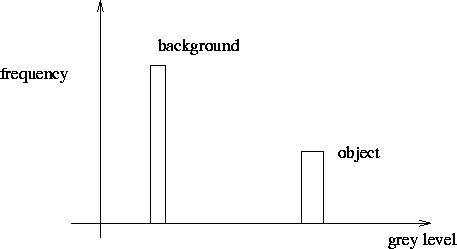But we have measurement noise. The histogram we end up seeing is the result of convolving the ideal' histogram with the probability distribution of the noise.If the grey levels of the object and the background are fairly close the influence of noise may result in the object only appearing as a shoulder' in the histogram.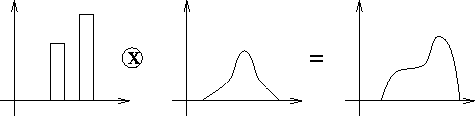In this case the histogram will no longer be bimodal. There will be no clear way of choosing the threshold. Repeated observations and averaging may help, but the spread of the histogram may be due to lighting variations or colour variations in the background and object.

## Analysis of Binary Images

We wish to determine various attributes of the objects in the scene with the aim of using these to identify the objects and to determine their position and orientation. We define the characteristic function of an object in an image to be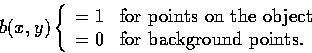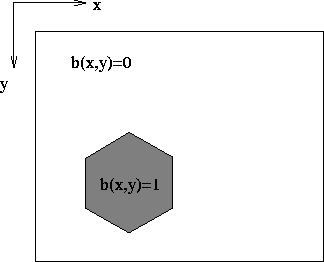Now consider the image to be continuous (that is, to have infinite resolution).

The area is given by the 0th moment of the object: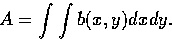The centre of mass, denoted by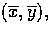is given by the 1st moments of the object: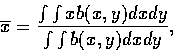andGenerally we use the axis of minimum inertia of the object to identify the orientation. This is the axis of least 2nd moment.We want to find the line for which the integral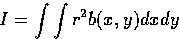is a minimum, where r is the perpendicular distance from (x,y) to the line we want to find (see figure 6).

The solution for the equation of this line is made more convenient if we parametrise the line using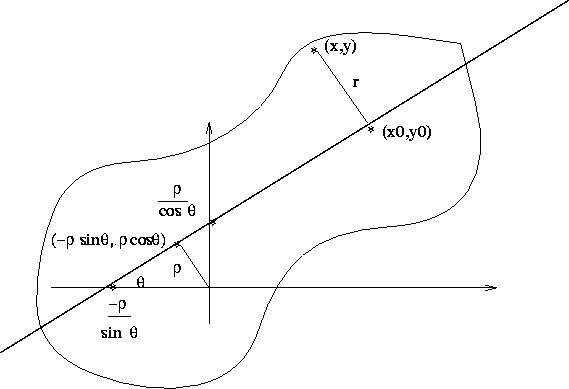Thus we have to solve for the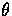and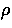that will give the axis of least second moment. Why do we specify the line like this?

Given this equation we can write parametric equations for points on the line as follows: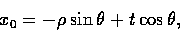and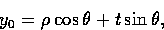where t is the distance along the line from the closest point to the origin.

Given a point (x,y) we need to find the closest point on the line so that we can calculate the distance r, which is given by (see figure 6)

r2 = (x - x0)2 + (y - y0)2.

We substitute for x0 and y0 to get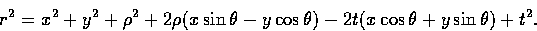What value of t minimizes this expression?

Differentiating with respect to t and equating the result to 0 gives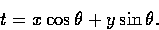So we substitute this back into a parametric equations for x0 and y0. This gives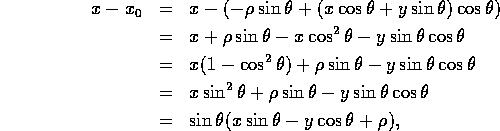and likewise for y - y0.

Thus r2 can be written asThis line is the locus of points for which r = 0. Hence, by parametrising the line in this manner we can obtain the distance from the line directly.

### Solving forandWe now want to find theandthat describe the line that minimizes distances of points in the object to that line, that is, that minimizes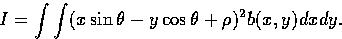We differentiate with respect toand set the resulting expression to 0, givingwhich is just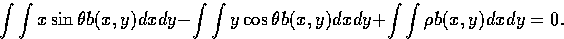Multiplying and dividing bygives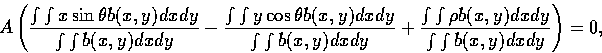and this is justwhere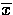,is the centre of mass. That is, the axis of minimum 2nd moment passes through the centre of mass.

We now have to find the value ofthat minimizes I. To do this, we change coordinates so that we are now working with respect to the centre of mass: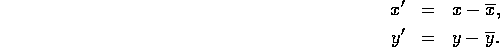This simplifies the equation of our line to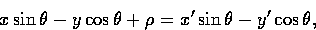and so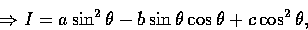where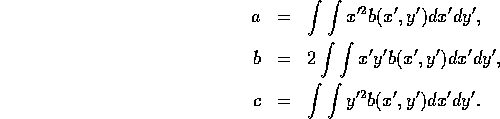The constants a, b, and c are called the second moments.

If we use the substitutions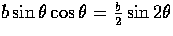and, then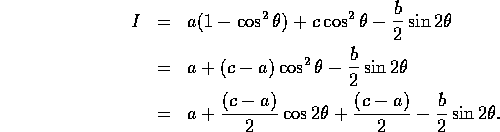Thus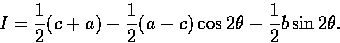We differentiate this expression with respect toand set the result to 0, obtaining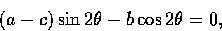which implies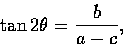unless b = 0 and a=c.

Thus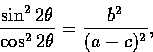which implies thatThis is just a quadratic in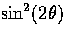, so we see that it has solution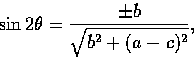and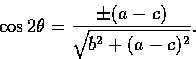When one chooses the positive solution, this minimizes I; when one chooses the negative solution, this maximizes I.

In the case that b=0 and a=c we see that I is unaffected by the direction of axis of orientation, that is, our object is rotationally symmetric.

The ratiogives us some idea of how rounded the object is. This ratio will be 0 for a line and 1 for a circle.Next: Discrete Binary Images Up: Computer Vision IT412 Previous: Measurements and Noise
Robyn Owens
10/29/1997